# Circular Functions: Equations & Examples

Lesson Transcript
Instructor: Betty Bundly

Betty has a master's degree in mathematics and 10 years experience teaching college mathematics.

In this lesson, we will learn how to define the basic trigonometric functions using a circle. In doing so, every angle in a circle will be paired with an ordered (x,y) point in the coordinate plane using the basic trigonometric functions.

## What Are Circular Functions?

You may have initially learned about the sine, cosine and tangent of an angle as the ratio of the sides of a right triangle. The sides that form the right angle are called legs, and the third side is called the hypotenuse. Given angle A, the three ratios are as follows:

• sinA = (length of side opposite angle A) / length of hypotenuse)
• cosA = (length of side adjacent to angle A) / (length of hypotenuse)
• tanA = (length of side opposite angle A) / (length of side adjacent to angle A) = sinA / cosA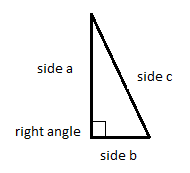The circular functions may be thought of as a way to extend these extremely useful mathematical relationships to any triangle. To visualize circular functions, we first start with a unit circle, or a circle with a radius equal to one unit of measurement.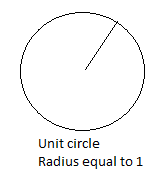In this circle, draw an x-y coordinate plane, with the origin at the center of the circle.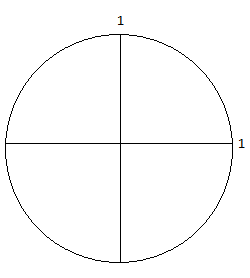Now, insert a right triangle in Quadrant I, so that the hypotenuse equals the radius of the circle, one leg of the right angle lies horizontally along the x-axis and the other leg is a vertical line that meets the hypotenuse lying on the circle. The angle will be formed by some length of the x-axis on one side and the hypotenuse on the other. The point of the circle where the hypotenuse and the vertical leg intersect is a rectangular coordinate point (x,y).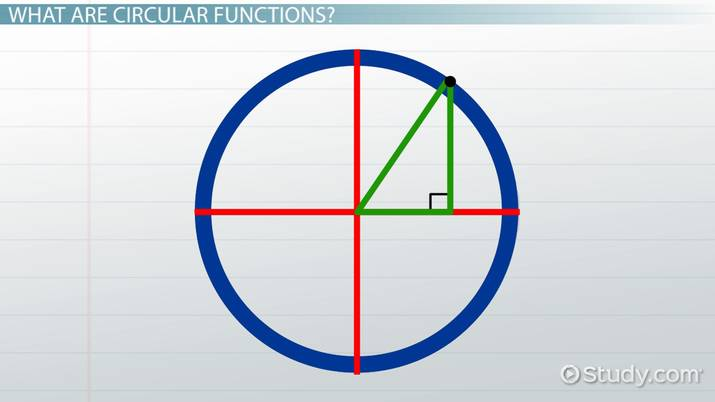An error occurred trying to load this video.

Try refreshing the page, or contact customer support.

Coming up next: Finding the Area of a Sector: Formula & Practice Problems

### You're on a roll. Keep up the good work!

Replay
Your next lesson will play in 10 seconds
• 0:00 What Are Circular Functions?
• 1:32 Using Trigonometry to…
• 3:41 Trigonometry & the…
• 4:48 Lesson Summary
Save Save

Want to watch this again later?

Timeline
Autoplay
Autoplay
Speed Speed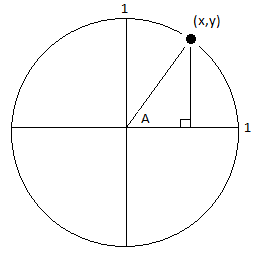## Using Trigonometry to Describe Points on a Circle

How does this x and y value translate into trigonometry? Since the hypotenuse is always equal to the radius of the circle, we call it r. Since the horizontal leg lies along the x-axis, we call it x. Since the vertical leg describes a position on the y-axis, we call it y. Using x, y, and r, we can now describe sinA, cosA as follows:

• SinA = y / r
• CosA= x / r

Now, to solve for x and y, multiply both sides of sinA = y / r by r and multiply cosA= x / r by r.

y = r * sinA

If we multiply both side of cosA = y / r by r, we obtain:

x = r * cosA

Now, as the hypotenuse moves counterclockwise around the circumference of the circle, a right angle can be formed this same way for every angle from 0 degrees to 360 degrees. Each of these angles can be described by the point (x,y) or (rcosA, rsinA).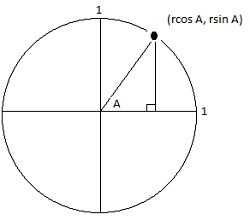To unlock this lesson you must be a Study.com Member.

### Register to view this lesson

Are you a student or a teacher?